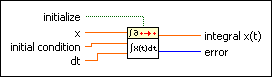# Integral x(t) PtByPt VI

## LabVIEW 2018 Help

Edition Date: March 2018
Part Number: 371361R-01
View Product InfoLabVIEW 2016 HelpLabVIEW 2017 HelpLabVIEW 2018 HelpLabVIEW 2019 HelpLabVIEW 2020 Help

Owning Palette: Integral & Differential PtByPt VIs

Requires: Full Development System

Performs the discrete integration on x. You must sample x at regular intervals of dt.

This VI is similar to the Integral x(t) VI.Note  By default, reentrant execution is enabled in all Point By Point VIs.initialize, when TRUE, initializes the internal state of the VI.x is an input data point.initial condition is the value used to calculate the integral for the first and second time.dt is the sampling interval and must be greater than 0. The default is 1.0. If dt is less than or equal to 0, the VI sets integral x(t) to 0 and returns an error.integral x(t) is the discrete integration of x.error returns any error or warning from the VI. You can wire error to the Error Cluster From Error Code VI to convert the error code or warning into an error cluster.

## Integral x(t) PtByPt Details

The Integral x(t) PtByPt VI calculates a definite integral. The value of integral x(t) is the area under the curve of the set of input data points between 0 and (n – 1)(dt).Note  You must pass the same value to initial condition on every call to this VI between one initialization of the VI and the next. The VI is not designed to integrate a curve of sample data points taken at irregular intervals. The VI returns erroneous values if you change initial condition on successive calls in an attempt to integrate a curve of sample data points taken at irregular intervals.